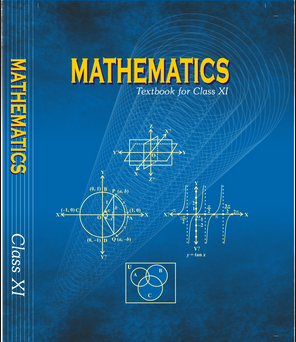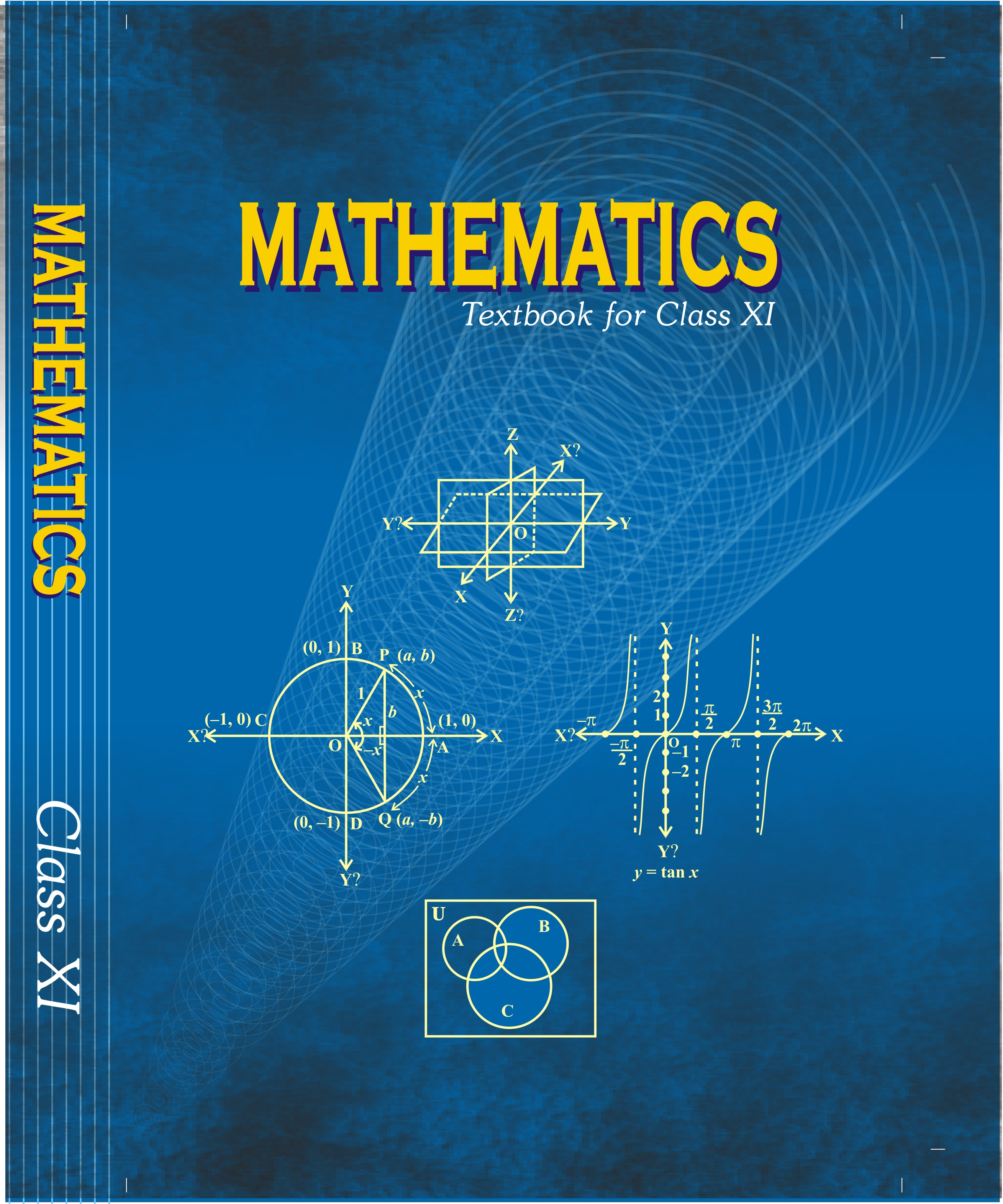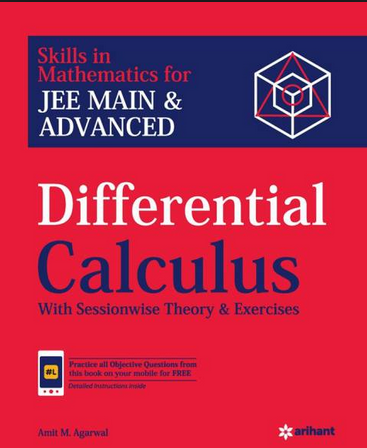# Limit , continuity and differentiability (Weightage 10%)   Share

### Topics from Limit , continuity and differentiability

• Graphs of simple functions, limits, continuty and differentiability. (55 concepts)
• Differentiation of the sum, difference, product and quotient of two functions (16 concepts)
• Differentiation of trigonometric, inverse trigonometric, logarithmic exponential, composite and implicit functions (16 concepts)
• Derivatives of order upto two (1 concepts)
• Apllications of derivatives monotonic, maxima and minima of functions of one variable, tangents and normals (34 concepts)
• Monotonic- increasing and decreasing functions (8 concepts)
• Rolle's and Lagrange's Mean Value theorems (5 concepts)
• Limits and their Importance (17 concepts)
• Differentiation (17 concepts)
• Continuity and Differentiability (13 concepts)
• Application of Derivatives (8 concepts)
• Monotonicity and Maxima-Minima of Functions (14 concepts)

### Important Books for Limit , continuity and differentiability

•••Exams
Articles
Questions